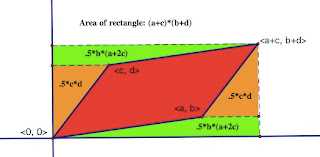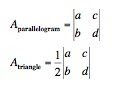## Thursday, May 29, 2008

### Schlope and PMAN

Mathematics is the art of giving the same name to different things. - Henri Poincare
So in this case it is not the quite the same name, but I have sort of "invented" some terminology. The idea of "schlope". Schlope is quite similar to slope. The slope of a line tells you how much to go up for every 1 that you move to the right. Schlope tells you what to multiply by for every one step to the right. Schlope is very important for exponential functions. Generally, in exponential situations we talk about the "rate of growth". If the rate of growth is 8% then the function that models that growth has "1.08 to the t" in it. That 1.08 is what I call "schlope". So, schlope is the number that you actually use in the formula.

In fact, you can create parallels for all your favorite formulas and constructs from linear functions. This parallel construction highlights another construct of mine that I like to call the hierarchy. The hierarchy is simply the recognition that the fundamental ways of putting numbers together come in an order. At the bottom level we have Addition and Subtraction. In the middle we have Multiplication and Division, and at the top powers, roots, and logarithms. Another teacher at my school refers to this as "PMAN" for powers, multiply, add, nothing. It is very helpful to think about PMAN when doing calculations with exponents and logs. If you are taking powers of powers, "P", then you "M"ultiply the powers. If you are "M"ultiplying powers with the same base then your "A"dd the exponents. If you are "A"dding different powers of the same base together then you do "N"othing.To move from Linear functions to Exponential functions you simply must move up in the hierarchy. Moving from repeatedly adding a number to the height every time to multiplying that height by some fixed number. The formulas can be written quite similarly:### Highlights Highlight

My wife and I wrote an article in Highlights magazine about factorial. You probably know the magazine from the doctors office. You can read the article here Big numbers at a banana-split bar but you miss all the pictures. Guess you will have to find it at the dentists office.

## Wednesday, May 28, 2008

### Are quadratics really that important

If I was a student who had just finished a course in Algebra I in most schools across America I think that I would think that the following were the most important things that we had done. I would think this because we had spent so much of our efforts on it.

• linear equations
• distributive law
• factoring
The problem with this list isn't so much what is on it, it is what isn't on it. It seems to me that we simply must include exponential functions and therefore also logarithms. I guess my question is, "Are quadratics really that important?"

The only way to add these topics in is to take other topics away. So what can go? Here is a partial list of topics that might be cut or reduced in the standard curriculum.

• completing the square
• conics
• rational root theorem
• long division of polynomials
Exponentials seem like such a natural topic, and one that could be made to support and build on linear equations so beautifully. I will give more of this idea when I write later about "schlope".

## Sunday, May 25, 2008

### Determinants as proportions

This is a continuation of a discussion of determinants started here. And there is more here.

Proportions

So if determinants are important how important are they and when can they be introduced reasonably. One place that they could possibly come up is in reference to proportions. As a reminder, a proportion is equation where two ratios are equal. One method to solve equations such as this is the Means-Extremes Property. This is more commonly known as “Cross-Multiplying”. I think I speak for many teachers that cross-multiplying is a “bane of existence”. Cross-multiplying is a rule that is often overused. It seems to quickly rise to the top of all students list of favorite methods so that whenever in doubt about how to proceed in a problem with fractions teachers often here the idea put forth that the correct method might include cross-multiplying. This is probably because few students really understand what this method does or why it works

Connections between determinants and proportions

So what is the connection between determinants and cross multiplying. Well
it can be seen from a variety of ways. First is in the formulas themselves. A
proportion has the form:after cross-multiplying we know thatthis last equation can be rewritten in terms of determinants asIn a proportion we are given that two fractions are the same. Each of those fractions can be thought of as vectors. Similar to the definition of slope as a fraction or as a vector. With this definition of the fraction we see that the two fractions will be equivalent if their vectors point in the same direction. If they point in the same direction then the area given by the determinant will be zero.

The advantage of this determinant method to solving proportions is that we eliminate the fractions from the problem. Cross-multiplying would only exist in determinants, where the rightly do play a role. Students would be less likely to misapply the idea of cross-multiplication to every situation with fractions. Determinants would be introduced earlier and their presentation of area would be well supported. Students would also have to have a clearer understanding of slope as a primary way of looking at fractions.

## Wednesday, May 14, 2008

### What is a determinant?

Determinants pros/cons
Determinants, for a teacher if I may borrow a British phrase, they are a bit of a sticky wicket. For my teaching, determinants have the qualities that I find makes a topic uninviting for students:

➢ The rules for manually calculating determinants seem arbitrary, and are nearly impossible to motivate.
➢ It is not clear what the determinant means. By which I mean it is not clear what “natural” meaning might be given to a the value of a determinant, in much the same way that slope can be imbued with the natural meaning of “rate of change”.

But determinants are also tempting:

➢ Cramer’s rule presents a powerful way to solve systems of equations
➢ Matrices are a vast generalization of number systems that have far reaching applications. Can we use determinants as a way to motivate the study of these objects?

When teaching we need motivations
The first question I ask about a topic, is what might lead someone to want to know this? Why have “slope” or a “determinant”. For motivation, slope benefits from the universality of change. A recurrent theme in mathematics education from algebra to calculus is the wide range of applications of the slope concept to measure change.
Each child must see the motivation in mathematics
History of math as a motivation
What can we use to motivate determinants? Often when I find myself struggling to motivate something I will study the historical motivations of a topic. For instance, the rules of probability are often well motivated by the story of the way Fermat and Pascal were lead to discuss a game that motivated many questions about probability.
The determinant’s history may not be as storied. It begins to late in the history of mathematics to have a wonderful story attached. Professional mathematicians, not interested lay practitioners brought it forth.
Connections/Generalizations of previous curriculum
To be a topic worthy of our student’s interest it must be made to represent something meaningful in their lives, in their experience, or in the larger life of the mind. Can we at least show that a curious soul would want to see what this is all about? We can only do this if the determinant can tell us something that our students find real. Rules that utilize determinants in part, such as Cramer’s rule, are inadequate for this job because the determinant is only part of the equation. Why not make the whole thing one giant new equation? We need to start with unadorned determinants to begin with.

In the rest of this article I will try to make the leap. I will try to motivate determinants and demystify them, and I will indicate some more uses for them. The more we understand about the determinants the more our students will understand them and find even more remarkable ways to use them.

Volume and Area
Volume and Area do provide a very concrete motivation. It is clear to a student that measuring the size of objects is a fundamental question of existence. There are two formulas for area that I would like to focus on. These are the standard the equations for the area of a parallelogram and the area of a triangle.

The inadequacies of some formulas for some areaThese formulas are absolutely correct and deeply flawed. The major drawback of these formulas is that they rely on a length that is not truly “part” of the shape. The height measurement would in reality be a difficult measurement to determine. How is it possible to determine that we are really are measuring an altitude?

Determinants provide another method to find area. In this version we must assume that the vertices have been given coordinates. Then it is possible to find vectors that represent the sides. These vectors not only encapsulate the length of the side but also the direction. It is therefore possible to find the area of a parallelogram or triangle from these measurements.

For instance, take the parallelogram shown below.In this case we can compute the vector from A to B is <> and from A to D is <>. Using these vectors we can compute the determinant and from that the area.Because we are interested in computing with vectors we can in general imagine that one of the corners of the parallelogram is at the origin. This gives us a figure like the one below.We can then compute the area of the parallelogram by finding the area of the rectangle and then subtracting the area of the green trapezoids and orange triangles.This shows that the area of the parallelogram can be computed from the vectors that define the parallelogram. Because triangles are half the size of a parallelogram we can compute the area of triangles similarly.Where and are the vectors that define two adjacent sides of our shapes.

This analysis can be extended to parallelepiped in three dimensions.

Next, we look at applying the determinant to problems involving proportions.